﻿ 数据结构与算法学习笔记 - Nemo

# 数据结构与算法学习笔记### 精通一个领域

1. Chunk it up 切碎知识点
• 庖丁解牛
• 脉络链接
2. Deliberate Practicing 刻意练习
3. Feedback 反馈

### 数据结构

• 一维：
• 基础：数组 array (string)，链表 linked list
• 高级：栈 stack，队列 queue，双端队列 deque，集合 set，映射 map (hash or map)，etc
• 二维：
• 基础：树 tree，图 graph
• 高级：二叉搜索树 binary search tree (red-black tree,AVL)，堆 heap，并查集 disjoint set，字典树 Trie，etc
• 特殊：
• 位运算 Bitwise，布隆过滤器 BloomFilter
• LRU Cache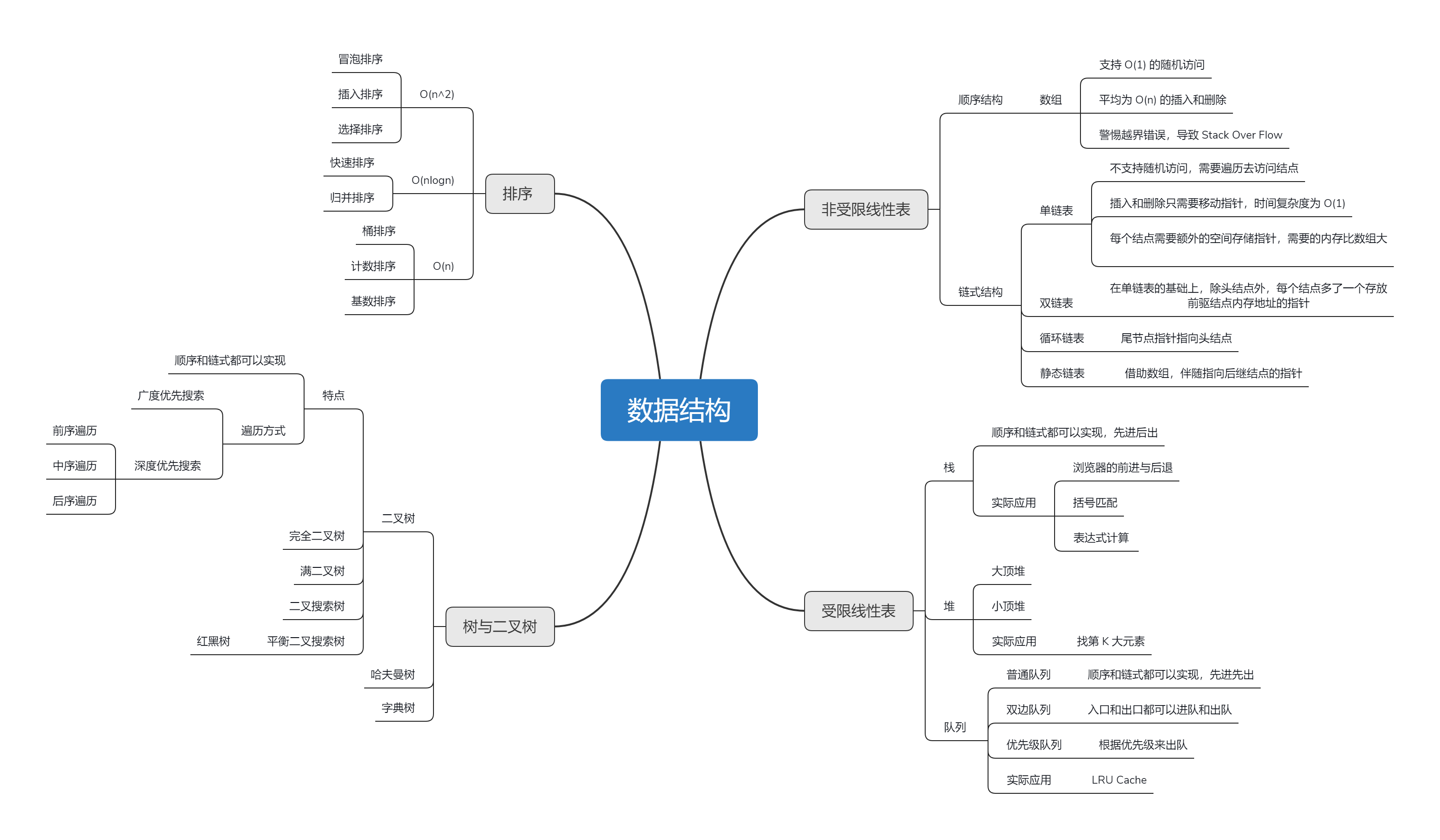### 算法

• if-else，switch ——> branch
• for，while loop ——> literation
• 递归 Recursion (Divide & Conquer ,Backtrace)

• 搜索 Search:深度优先搜索 Depth first search, 广度优先搜索 Breadth first search，A*,etc

• 动态规划 Dynamic Programming

• 二分查找 Binary Search

• 贪心 Greedy

• 数学 Math，几何 Geometry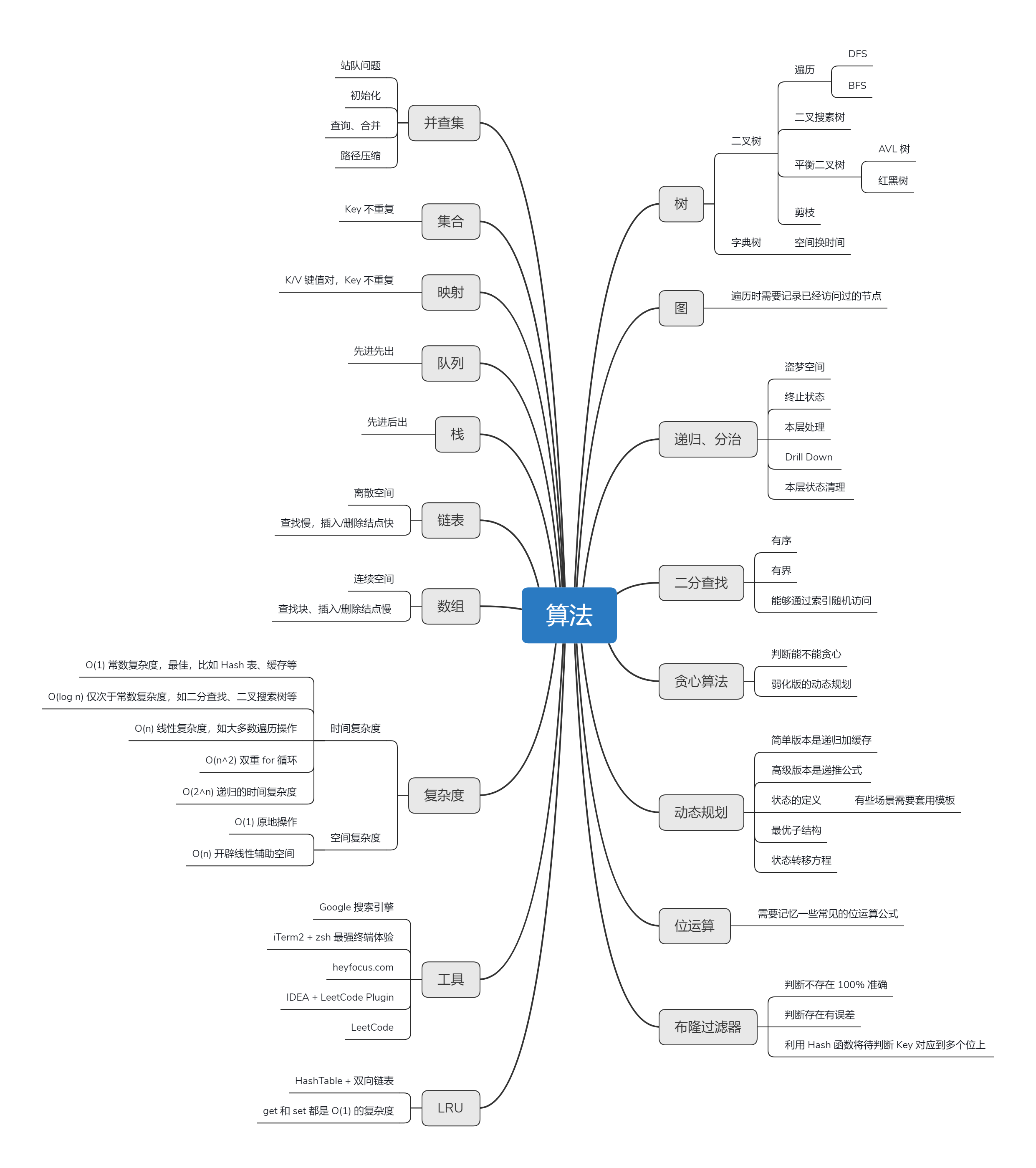### 职业化运动

• 基本功是区别业余和职业化选手的根本

• 基础动作的分解训练和反复练习 ——> 最大的误区

### Deliberate Practicing

• 刻意练习 —— 过遍数（五毒神掌）
• 练习缺陷、弱点地方

### Feedback

• 即时反馈

• 主动型反馈（自己去找）
• 高手代码（Github，Leetcode，etc）
• 第一视角直播
• 被动式反馈（高手给你指点）
• code review

### 切题四件套

• Clarification
• Possible Solutions
• compare (time/space)
• optimal （加强）
• Coding（多写）
• Test cases

### 五毒神掌

#### 第一遍

• 五分钟：读题 + 思考
• 直接看解法：多看几种，比较解法优劣
• 背诵、默写好的解法

#### 第二遍

• 马上自己写 ——> Leetcode提交
• 多种解法比较、体会 ——> 优化！

#### 第三遍

• 过了一天后，再重复做题
• 不同解法的熟练程度——>专项练习

#### 第四遍

• 过了一周后：反复回来练习相同的题目

• 面试前一周恢复性训练

### 小结

• 职业训练：拆分知识点、刻意练习、反馈
• 五步刷题法
• 做算法题最大的误区：只做一遍

• 上国际站看解答

## 时间复杂度和空间复杂度

### Big O notation

• O(1)：Constant Complexity 常数复杂度
• O(log n)：Logarithmic Complexity 对数复杂度
• O(n)：Linear Complexity 线性时间复杂度
• O(n^2)：N Square Complexity 平方
• O(n^3)：N Square Complexity 立方
• O(2^n)：Exponential Growth 指数
• O(n!)：Factorial 阶乘

#### O(1)

# 看代码执行了1次
n = 1000
print(n) # 不管n为多少，print只执行一次

# 代码执行了三次，O(3)，还是O(1)
m = 1000
print(m)
print(m)
print(m)


#### O(N)

# 代码执行了n次
for i in range(n):
print('执行了：',i)


#### O(N^2)

for i in range(n):
for j in range(m):
print('执行了:',i+j)


#### O(log(n))

# 这个需要重写
for i*i in range(n):
print(i)



#### O(k^n):

def fib(n):
if n <= 2 :
return n
else:
return fib(n-1) + fib(n-2)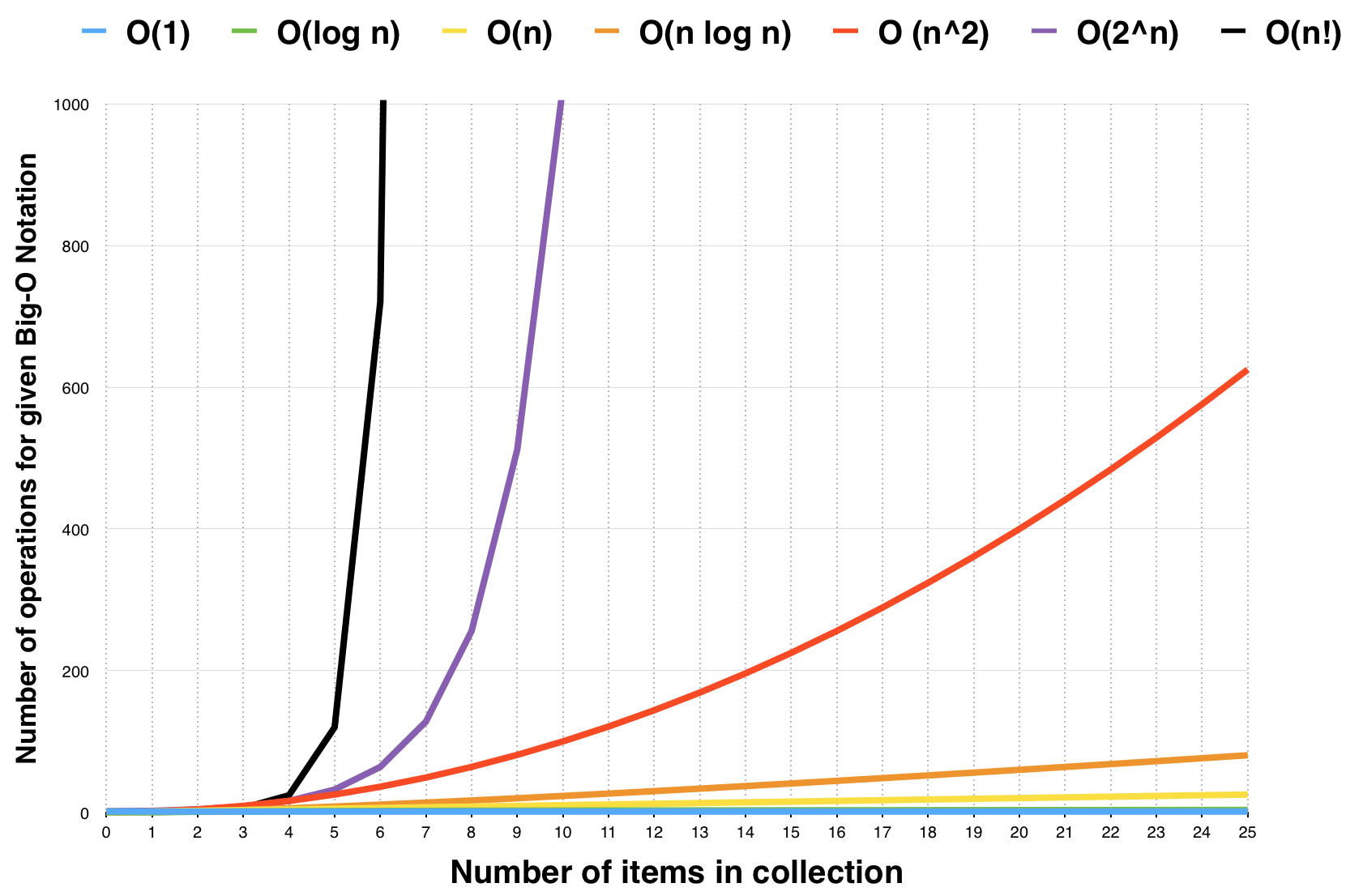#### 计算：1+2+…+n：

• 方法一：
# O(n)
y = 0
for i in range(1,n+1):
y += i

• 方法二：
# O(1)
y = n * (n+1) / 2


### 更复杂的情况：递归

#### Fib:0,1,1,2,3,5,8,13,21,…

• F(n) = F(n-1) + F(n-2)

• 最简单的写法，直接用递归

def fib(n):
if n <= 2:
return n
return fib(n-1)+fib(n-2)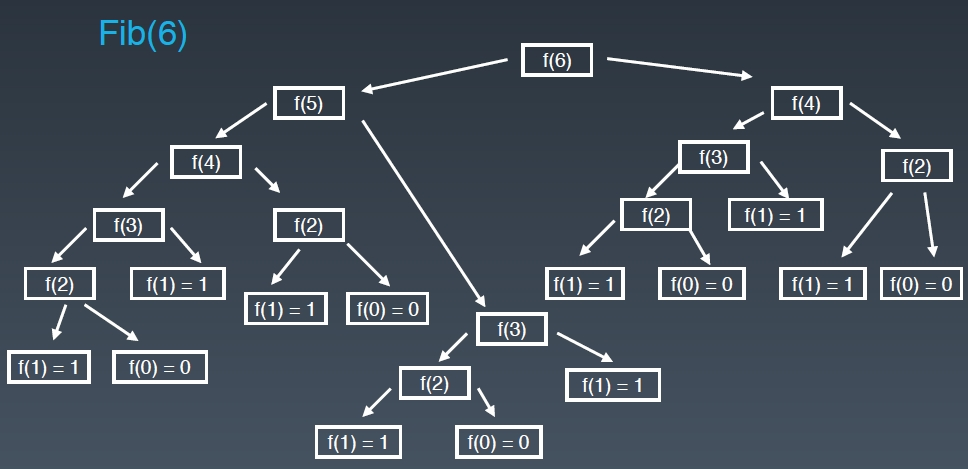• 两个’灾难’：
1. 每展开一层，运行的节点数就是上层的两倍，按指数级递增
2. 存在重复计算的节点

#### Master Theorem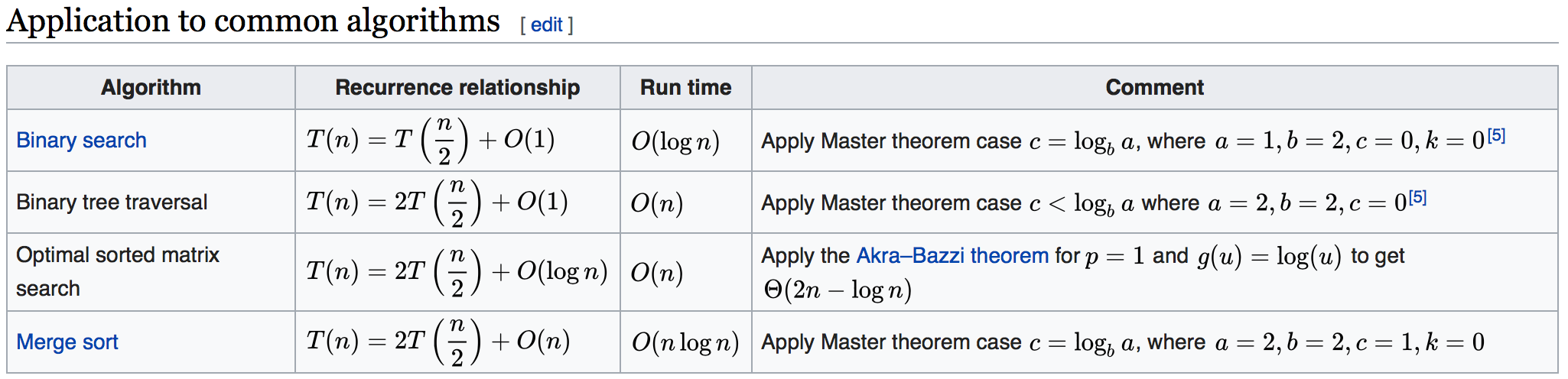• 一维数组二分查找：每次排除一半，故为O(log n)
• 二叉树的遍历：可以理解成每个节点被访问且仅访问一次，故为O(n)
• 二维矩阵的二分查找：O(n)
• 归并排序：O(n logn)

#### 思考

1. 二叉树遍历：前序、中序、后序的时间复杂度是多少？
• O(n)
1. 图的遍历：时间复杂度是多少？
• O(n)
1. 搜索算法：DFS、BFS的时间复杂度是多少？
• O(n)

1-3中因为每个节点访问且只访问一次

1. 二分查找：时间复杂度是多少？
• O(log n)

### Array

#### 创建

Java、C++： int a

Python：list=[]

JavaScript：let x=[1,2,3] # 可以放入初始值

#### 结构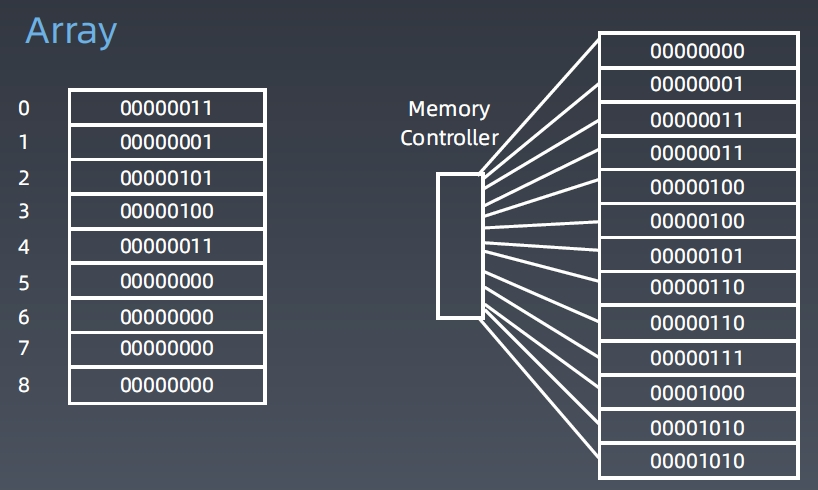• 可以随机访问任何一个元素，时间为O(1)。

• 插入、删除元素，时间为O(n)。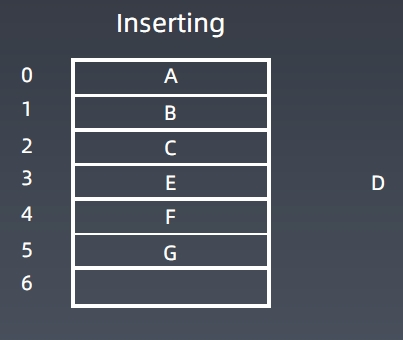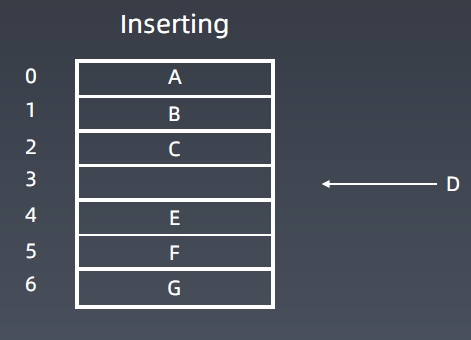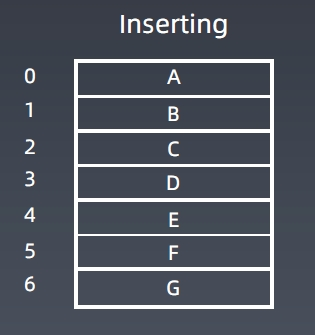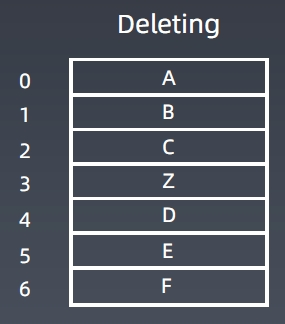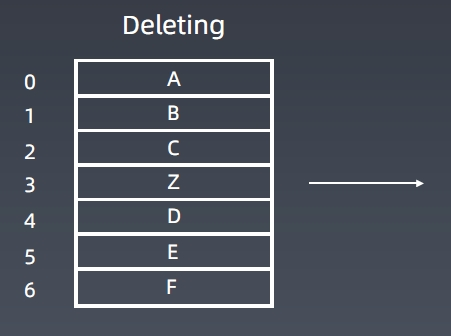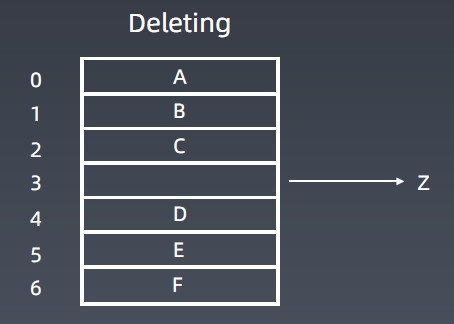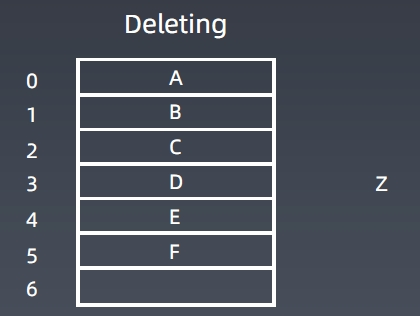prepend O(1)
append O(1)
lookup O(1)
insert O(n)
delete O(n)

#### 结构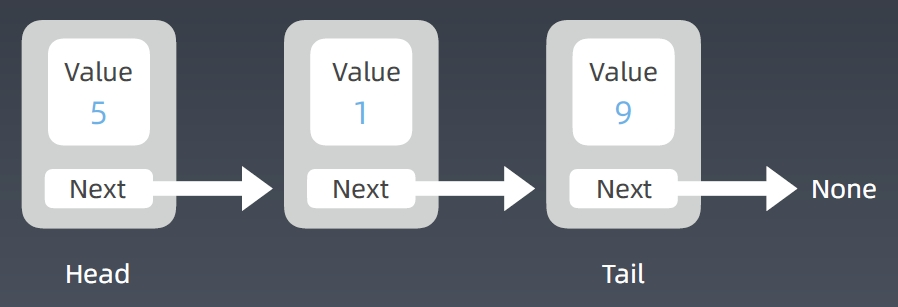• 只有一个指针，称之为单链表
• 多加一个前项指针，称之为双链表 Double Linked List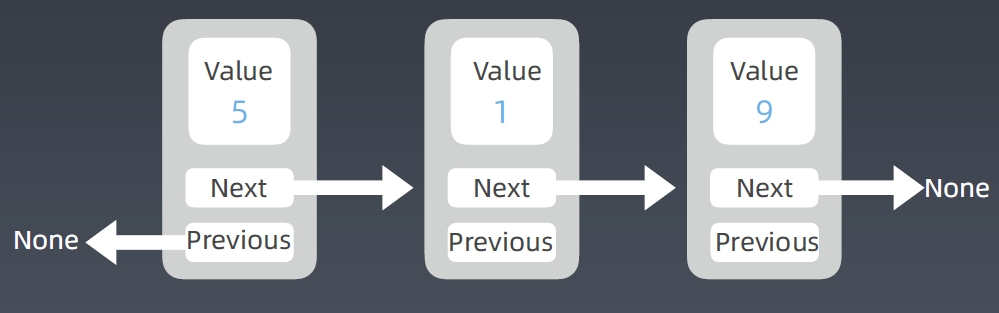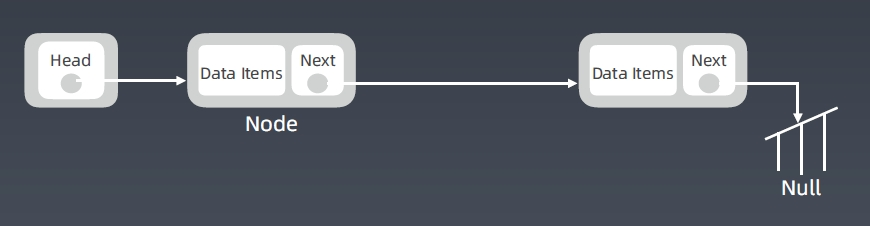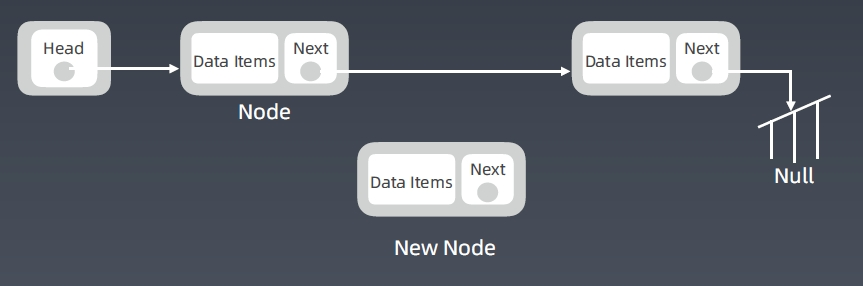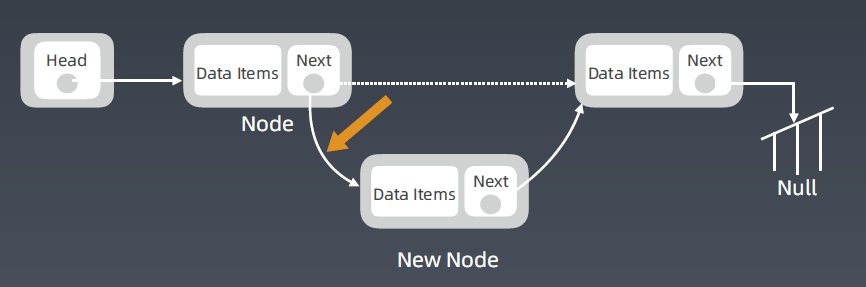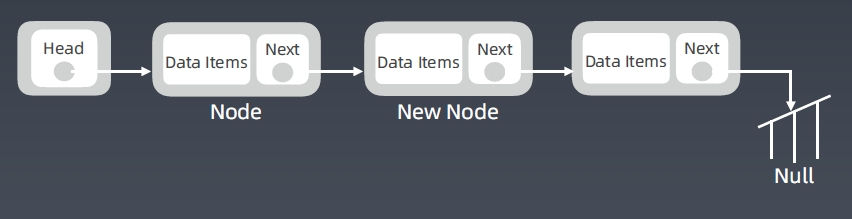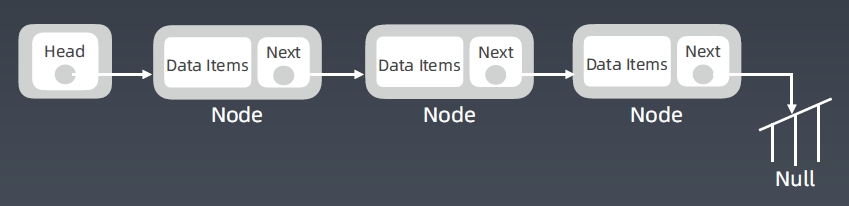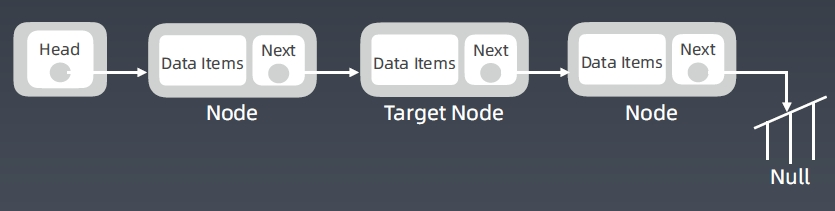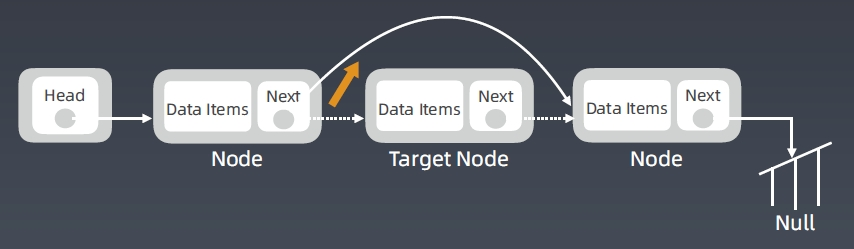prepend O(1)
append O(1)
lookup O(n)
insert O(1)
delete O(1)

#### 链表的优化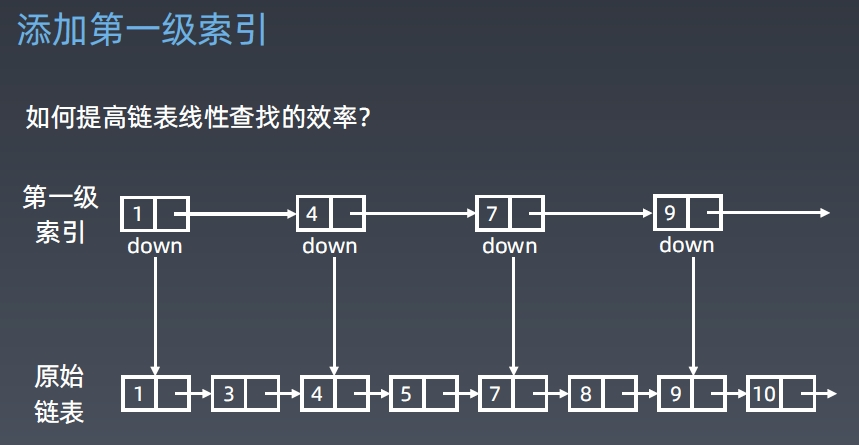### 跳表 Skip List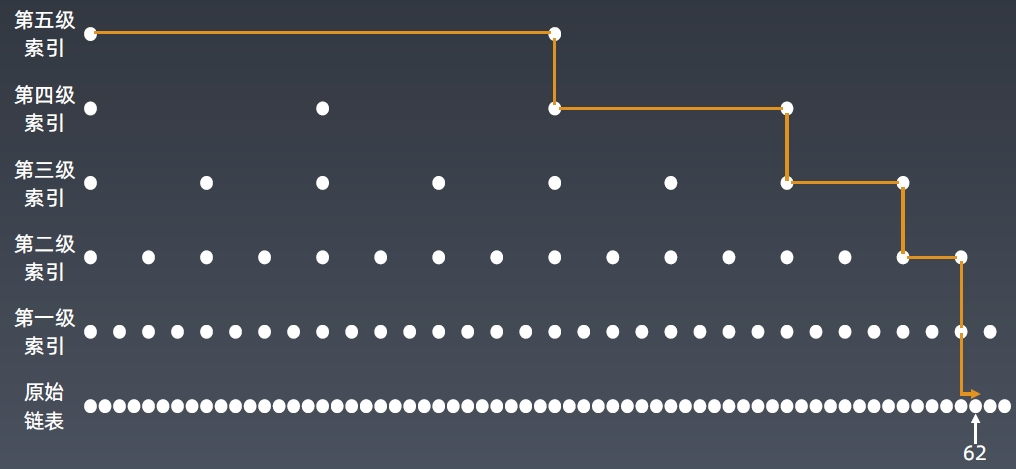#### 跳表查询的时间复杂度分析

n/2、n/4、n/8、第k级索引结点的个数就是

\frac{n}{2^k}

\frac{n}{2^h}=2

，从而求得

h=\log _2n-1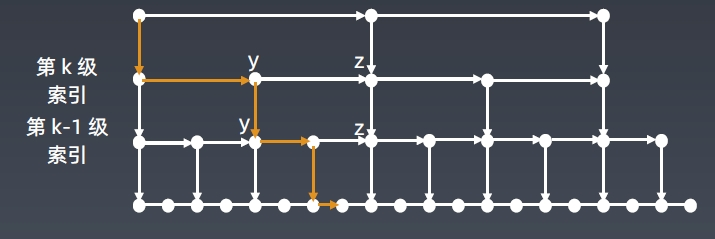#### 现实中跳表的形态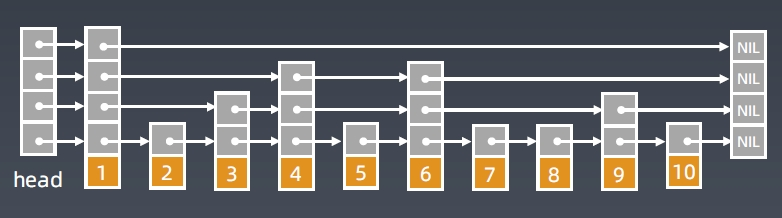#### 跳表的空间复杂度分析

\frac{n}{2},\frac{n}{4},\frac{n}{8},…,8,4,2

\frac{n}{3},\frac{n}{9},\frac{n}{27},…,9,3,1

#### 工程中应用

https://www.jianshu.com/p/b1ab4a170c3c
https://leetcode-cn.com/problems/lru-cache

Redis – Skip List

skiplist.html
https://www.zhihu.com/question/20202931

### 小结

• 数组、链表、跳表的原理和实现
• 三者的时间复杂度、空间复杂度
• 工程运用
• 跳表：升维思想 + 空间换时间

### Stack### Queue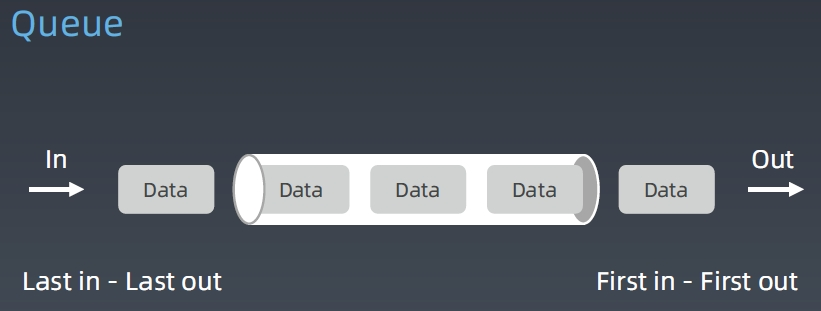### Deque: Double-End Queue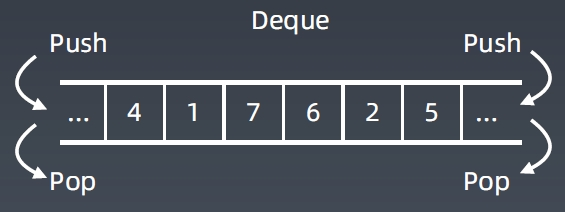• 简单理解：两端可以进出的 Queue
• 插入和删除都是 O(1) 操作，查询是 O(n)

### Stack、Queue、Deque 的工程实现

• 是使用一个数组进行模拟

• 如何查询接口信息？如何使用？

• 代码示例

Stack<Integer> stack = new Stack<>();
stack.push(1);
stack.push(2);
stack.push(3);
stack.push(4);
System.out.println(stack);
System.out.println(stack.search(4));
stack.pop();
stack.pop();
Integer topElement = stack.peek();
System.out.println(topElement);
System.out.println(" 3的位置 " + stack.search(3));

Queue<String> queue = new LinkedList<String>();
queue.offer("one");
queue.offer("two");
queue.offer("three");
queue.offer("four");
System.out.println(queue);
String polledElement = queue.poll();
System.out.println(polledElement);
System.out.println(queue);
String peekedElement = queue.peek();
System.out.println(peekedElement);
System.out.println(queue);
while(queue.size() > 0) {
System.out.println(queue.poll());
}

• Deque
Deque<String> deque = new LinkedList<String>();
deque.push("a");
deque.push("b");
deque.push("c");
System.out.println(deque);
String str = deque.peek();
System.out.println(str);
System.out.println(deque);
while (deque.size() > 0) {
System.out.println(deque.pop());
}
System.out.println(deque);


### Priority Queue

• 插入操作：O(1)

• 取出操作：O(logN) – 按照元素的优先级取出，每次取是取优先级最高的。

• 底层具体实现的数据结构较为多样和复杂：heap、bst、treap

[Java 的 PriorityQueue](https://docs.oracle.com/javase/10/docs/api/java/util/
PriorityQueue.html)

### Python相关代码

class Stack:

def __init__(self):
self.items = ['x', 'y']

def push(self, item):
self.items.append(item)

def pop(self):
self.items.pop()

def length(self):
return len(self.items)

class Queue:

def __init__(self):
self.queue = []

def enqueue(self, item):
self.queue.append(item)

def dequeue(self):
if len(self.queue) < 1:
return None
return self.queue.pop(0)

def size(self):
return len(self.queue)


### 复杂度分析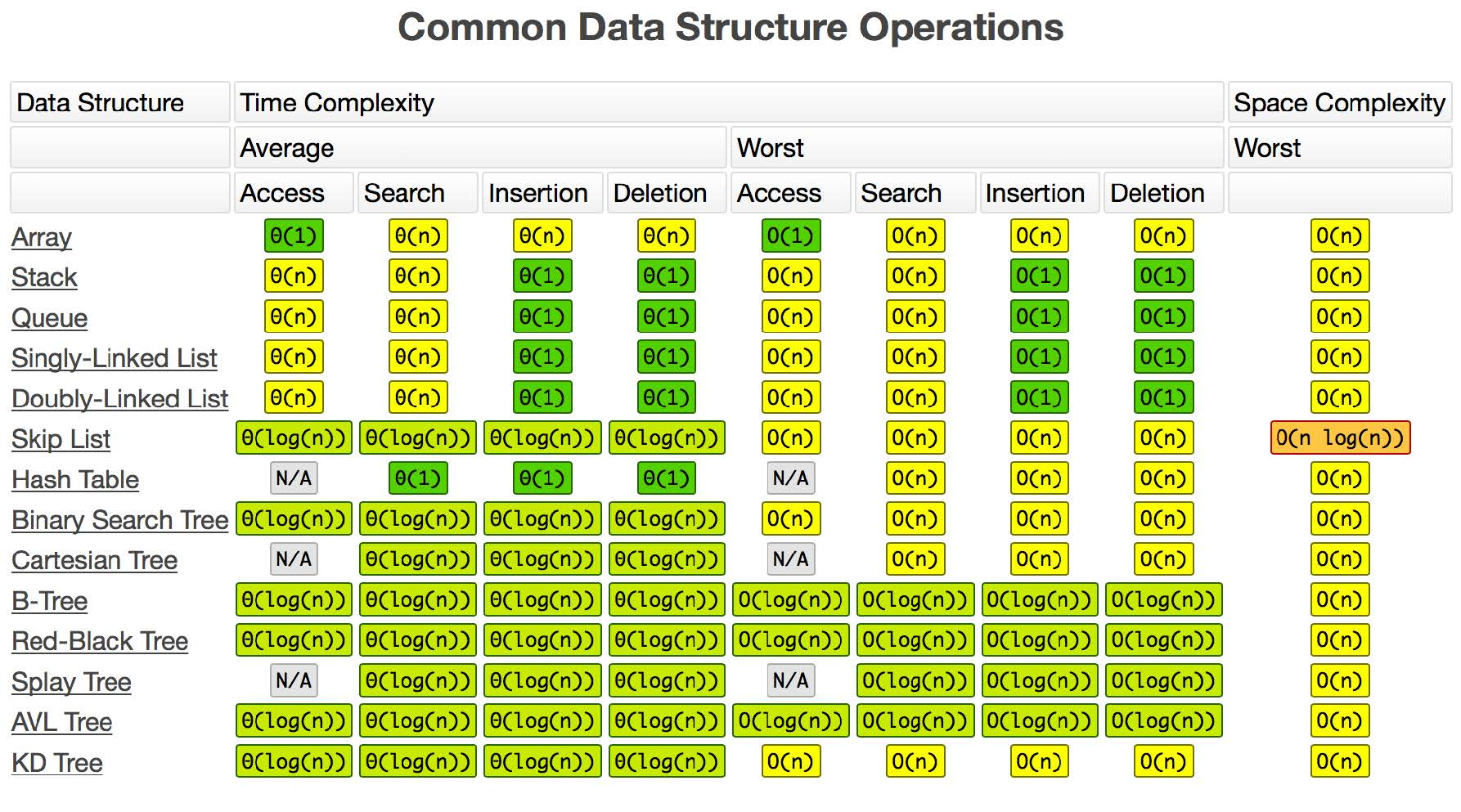### 小结

1. Stack、Queue、Deque 的原理和操作复杂度
2. PriorityQueue 的特点和操作复杂度
3. 查询 Stack、Queue、Deque、PriorityQueue 的系统接口的方法

### Hash table

• 哈希表（Hash table），也叫散列表，是根据关键码值（Keyvalue）而直接进行访问的数据结构。
• 它通过把关键码值映射到表中一个位置来访问记录，以加快查找的速度。
• 这个映射函数叫作散列函数（Hash Function），存放记录的数组叫作哈希表（或散列表）

Python – Hash Table

#### 工程实践

• 电话号码簿
• 用户信息表
• 缓存（LRU Cache）
• 键值对存储（Redis）

#### Hash Function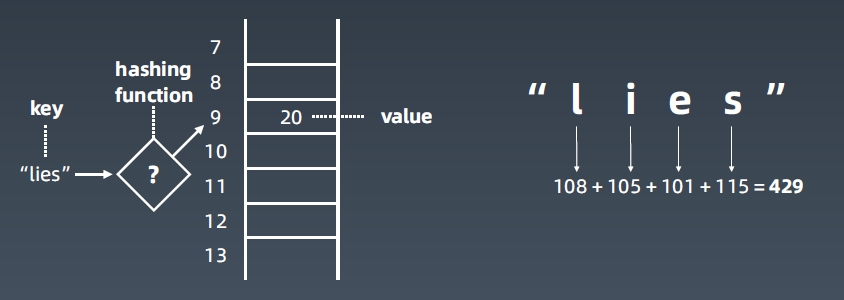#### Hash Collisions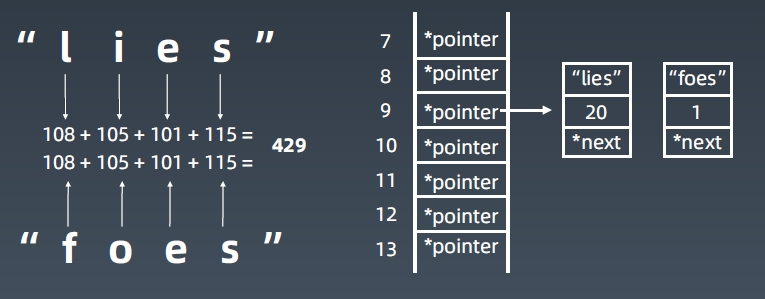• 发生哈希碰撞解决办法就是在碰撞的地方存一个链表——拉链式解决冲突法

• Hash表查询O(1)，最坏发生碰撞，退化为O(n)#### 复杂度分析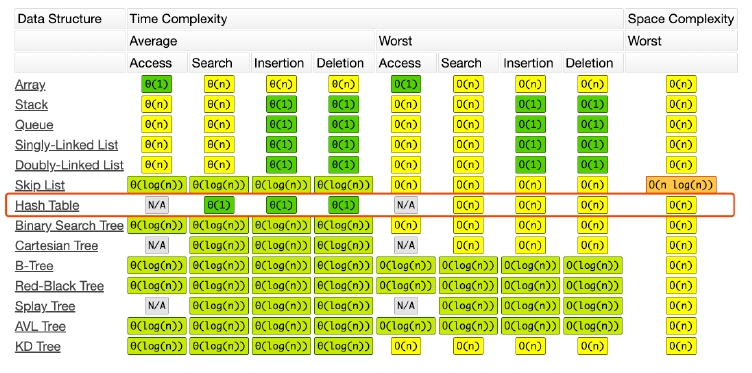### Map、Set

• Map : key-value对，key不重复
• Set : 不重复元素的集合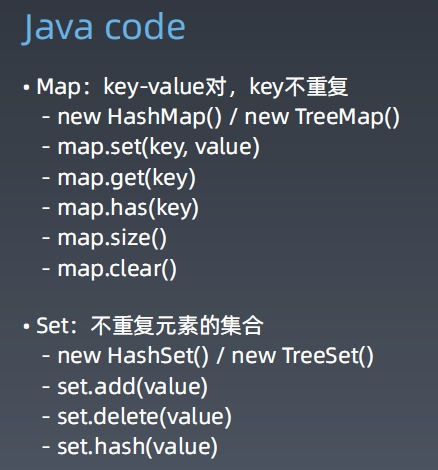list_x = [1,2,3,4]

map_x = {
'jack':100,
'张三':80,
'selina':90,
...
}

set_x = {'jack','selina','Andy'}
set_y = set(['jack','selina','jack'])

• Java set classes:

TreeSet, HashSet,

ConcurrentSkipListSet, CopyOnWriteArraySet, EnumSet, JobState

https://docs.oracle.com/en/java/javase/12/docs/api/java.base/java/util/Set.html

• Java map classes:

HashMap, Hashtable, ConcurrentHashMap

https://docs.oracle.com/en/java/javase/12/docs/api/java.base/java/util/Map.html

#### 复杂度分析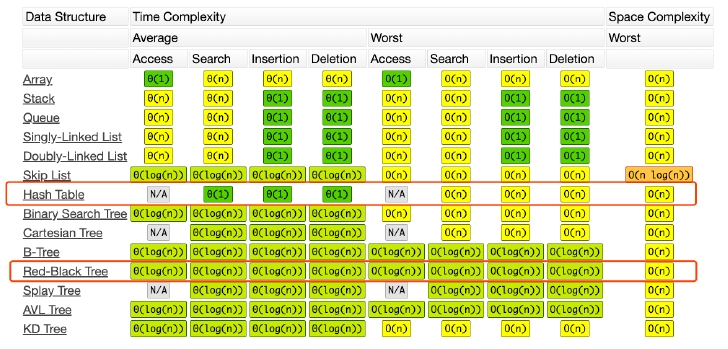### 链表等一维结构### 树 Tree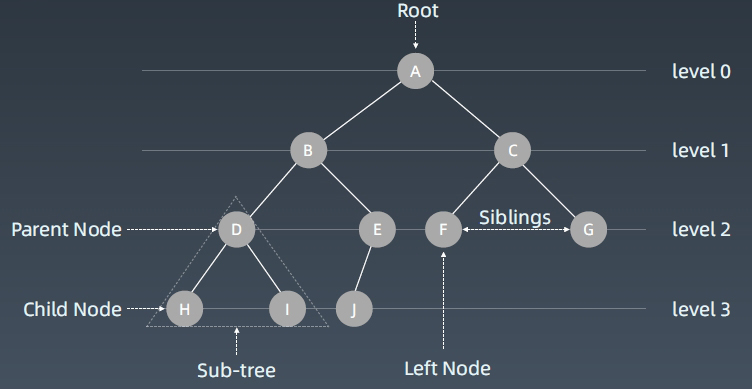#### 图 Graph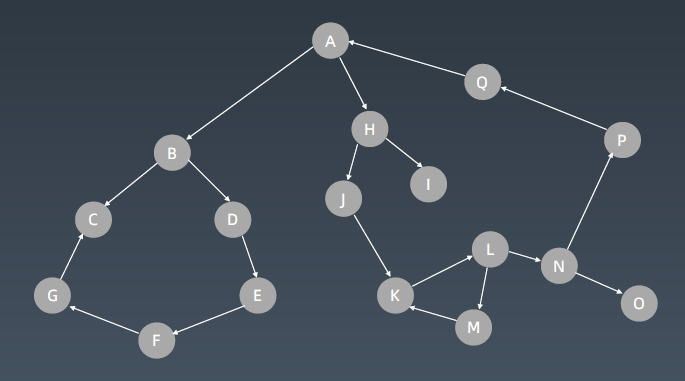Tree 是特殊化的 Graph

#### 二叉树 Binary Tree——最常见的树结构

class TreeNode:
def __init__(self,val):
self.val = val
self.left,self.right = None,None


“C++

struct TreeNode {
int val;
TreeNode *left;
TreeNode *right;
TreeNode(int x) : val(x), left(NULL), right(NULL) {}
}

<pre><code class="line-numbers">“java
public class TreeNode {
public int val;
public TreeNode left, right;
public TreeNode(int val) {
this.val = val;
this.left = null;
this.right = null;
}
}

#### 二叉树遍历 Pre-order/In-order/Post-order

1. 前序 (Pre-order)：根-左-右
2. 中序 (In-order)：左-根-右
3. 后序(Post-order)：左右根

def preorder(self,root):
if root:
self.traverse_path.append(root.val)
self.preorder(root.left)
self.preorder(root.right)

def inorer(self,root):
if root:
self.inorder(root.left)
self.traverse_path.append(root.val)
self.inorder(root.right)

def postorder(self,root):
if root:
self.postorder(root.left)
self.postorder(root.left)
self.traverse_path.append(root.val)


#### 二叉搜索树 Binary Search Tree

1. 左子树上所有结点的值均小于它的根结点的值；

1. 右子树上所有结点的值均大于它的根结点的值；

2. 以此类推：左、右子树也分别为二叉查找树。 （这就是 重复性！）

#### 二叉搜索树常见操作

1. 查询
2. 插入新结点（创建）
3. 删除：
• 删除叶结点很简单；
• 删除某个根节点：找到它周围最接近它的值的节点替换掉它，往往是找到刚刚大于这个根节点的节点值

#### 复杂度分析#### 思考

1. 结点的定义就是以递归方式进行；

2. 重复性（自相似性）

二叉搜索树：左子树小于根节点，右子树大于根节点，且左右子树具有相同特征

1. 节点的定义
2. 重复性（自相似性）

def preorder(self, root):
if root:
self.traverse_path.append(root.val)
self.preorder(root.left)
self.preorder(root.right)
def inorder(self, root):
if root:
self.inorder(root.left)
self.traverse_path.append(root.val)
self.inorder(root.right)
def postorder(self, root):
if root:
self.postorder(root.left)
self.postorder(root.right)
self.traverse_path.append(root.val)


### 递归-Recursion

• 递归 – 循环
• 通过函数体来进行的循环
1. 从前有个山
2. 山里有个庙
3. 庙里有个和尚讲故事
4. 返回1• 向下进入到不同梦境中；向上又回到原来一层——归去来兮
• 通过声音同步回到上一层
• 每一层的环境和周围的人都是一份拷贝、主角等几个人（想当于函数里面的参数）穿越不同层级的梦境（发生和携带变化）

n！= 1 * 2 * 3 * … * n

def Factorial(n):
if n <= 1:
return 1
return n * Factorial(n — 1)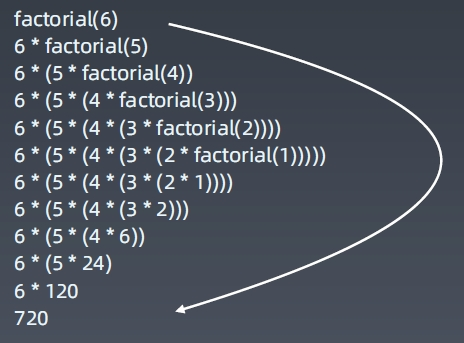#### 递归的Python模版

def recursion(level, param1, param2, ...):

# recursion terminator 递归终止条件，否则会无限递归造成死循环
if level > MAX_LEVEL:
process_result
return

# process logic in current level 处理当前层逻辑
process(level, data...)

# drill down 下探到下一层
self.recursion(level + 1, p1, ...)

# reverse the current level status if needed 清理当前层


#### 递归的Java模版

public void recur(int level, int param) {
// terminator
if (level > MAX_LEVEL) {
// process result
return;
}

// process current logic
process(level, param);

// drill down
recur( level: level + 1, newParam);

// restore current status
}


### 思维要点

1. 不要人肉进行递归（最大误区）
2. 找到最近最简方法，将其拆解成可重复解决的问题（重复子问题）
3. 数学归纳法思维

### 递归状态树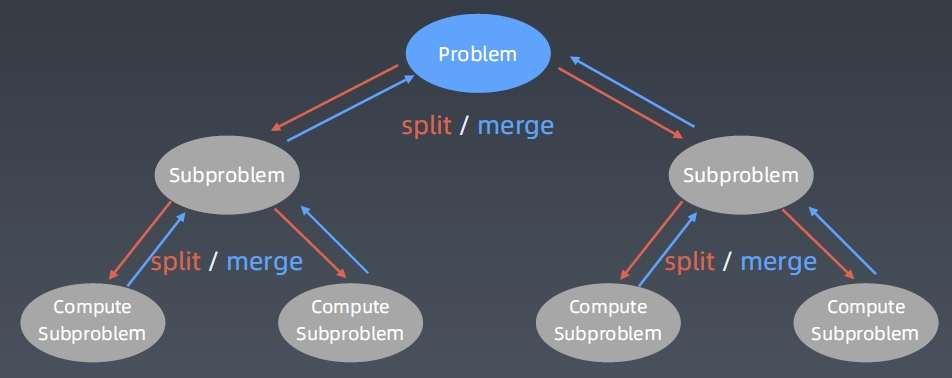### 分治 Divide & Conquer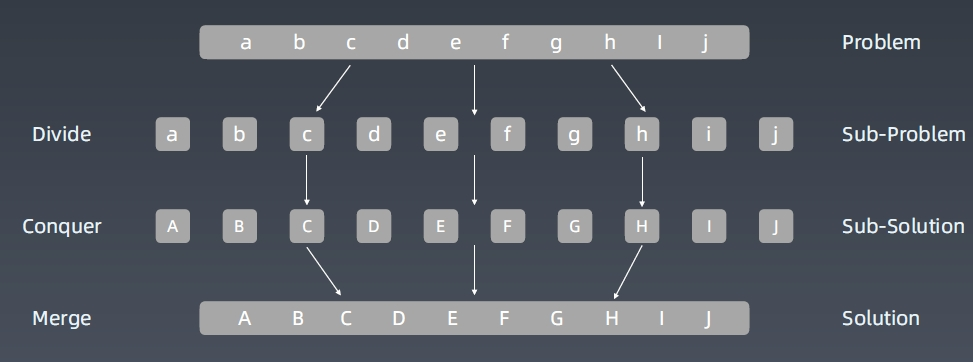### 分治代码模版

def divide_conquer(problem, param1, param2, ...):

# recursion terminator
if problem is None: # 对于分治来说一般是到达了叶子节点
print_result
return

# prepare data
data = prepare_data(problem)
subproblems = split_problem(problem, data)

# conquer subproblems
subresult1 = self.divide_conquer(subproblems, p1, ...)
subresult2 = self.divide_conquer(subproblems, p1, ...)
subresult3 = self.divide_conquer(subproblems, p1, ...)
...

# process and generate the final result
result = process_result(subresult1, subresult2, subresult3, …)

# revert the current level states


### 回溯 Backtracking

• 找到一个可能存在的正确的答案；
• 在尝试了所有可能的分步方法后宣告该问题没有答案。

### 遍历搜索

Python中树的节点定义：

class TreeNode:
def __init__(self,val):
self.val = val
self.left,self.right = None,None


### 搜索-遍历

• 每个节点都要访问
• 每个节点仅仅要访问一次——主要是为了效率
• 对于节点的访问顺序不限
• 深度优先：depth first search
• 优先级优先

#### DFS算法——通过递归、栈实现

##### 遍历顺序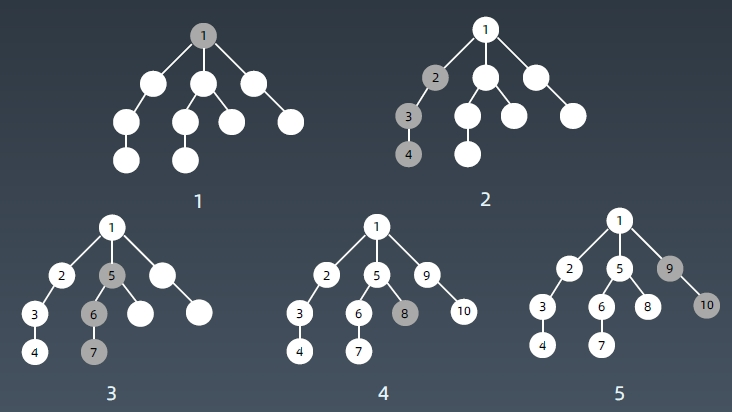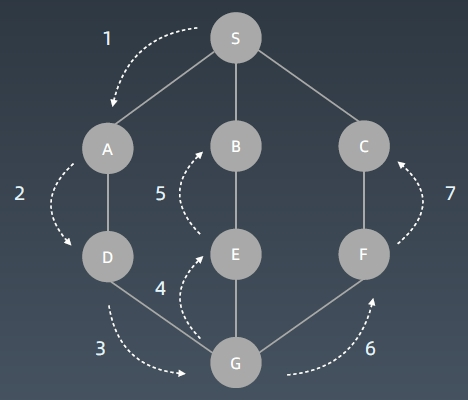##### DFS代码模版:
visited = set()

def dfs(node):
if node in visited:
return

# process current node
# ...# logic here
dfs(node.left)
dfs(node.right)


DFS递归方式代码模版:

visited = set()

def dfs(node, visited):
if node in visited: # terminator
return

# process current node here.
...
for next_node in node.children():
if not next_node in visited:
dfs(next_node, visited) # 递归调用的好处是不用等循环跑完，就已经进入下一层了


def DFS(self, tree):

if tree.root is None:
return []

visited, stack = [], [tree.root]

while stack:
node = stack.pop()

process (node)
nodes = generate_related_nodes(node)
stack.push(nodes)

# other processing work
...


#### BFS算法-通过队列实现

##### 遍历顺序BFS与DFS遍历顺序比较：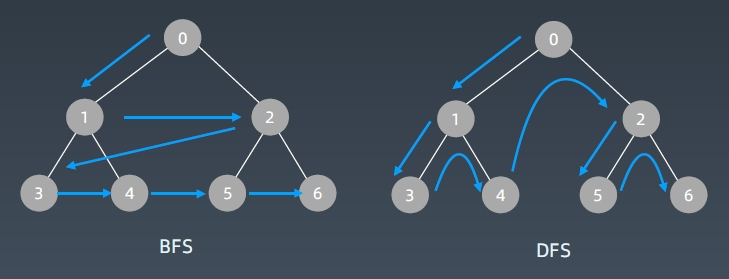##### BFS队列实现代码：
def BFS(graph, start, end):

queue = []
queue.append([start])

while queue:
node = queue.pop()

process(node)
nodes = generate_related_nodes(node)
queue.push(nodes)

# other processing work
...


### 实战题目

Coin Change 特别版本：

#### 322. 零钱兑换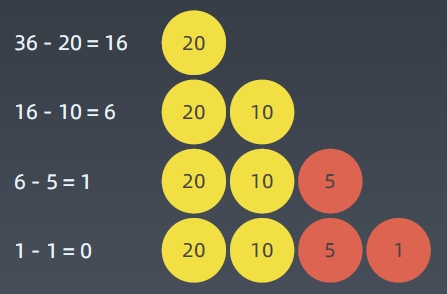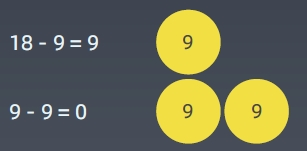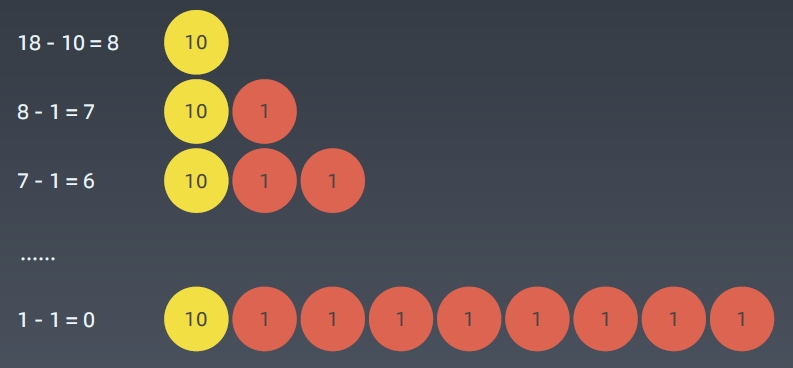#### 适用贪心算法的场景Nemo版权所有丨如未注明，均为原创丨本网站采用BY-NC-SA协议进行授权，转载请注明转自：https://nemo.cool/428.html
# #### 推荐文章

•Soldier

好棒 我也走一波

2年前 回复
•kanghaov博主

@Soldier： 嗯嗯，可以可以

2年前 回复
•jackie

牛，就是少了实战题目部分，包括题解就完美了

6月前 回复 提示 精通一个领域 Chunk it up 切碎知识点 庖丁解牛 脉络链接 Deliberate Practicing 刻意练习 Feedback 反馈 数据结构 一维： 基础：数组 array (string)，链…

2019-10-27